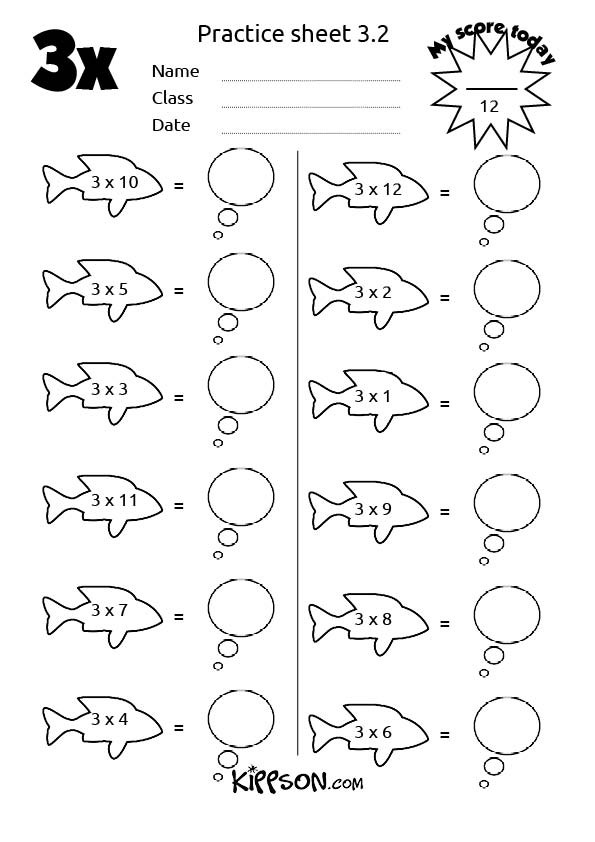Search

# Turbo boost your three times table

and check out the little trick for knowing if a number is divisible by 3Struggling to learn the three times table?

Print out our free worksheets - practice is the key.The three times table can be quickly and pleasingly written out in a logical grid format. If you love patterns check out this video.

Freaked out by division? The three times tables is really interesting - try this little trick:

To test if a number is divisible by 3, just add together its digits.

The answer will ALWAYS be 3, 6 or 9

Here - check this example out

Is 24 divisible by 3?

Add together its digits (2 + 4 = 6)

So yes, 24 IS divisible by 3

Is 50 divisible by 3?

Add together its digits (5 + 0 = 5)

No, the answer needs to by 3, 6, or 9

50 is NOT divisible by 3

Is 78 divisible by 3?

Add together its digits (7 + 8 = 15 This is a two digit number, so keep adding: 1 + 5 = 6)

Yes! This 78 is divisible by 3

There is a similar test for the six times table, but there is a small yet important difference: ALL the numbers in the 6 times table are EVEN numbers. So if you are starting with an odd number, it will NOT be divisible by 6 (but it might be divisible by 3)

For more worksheets, ideas and resources visit www.kippson.com

See All